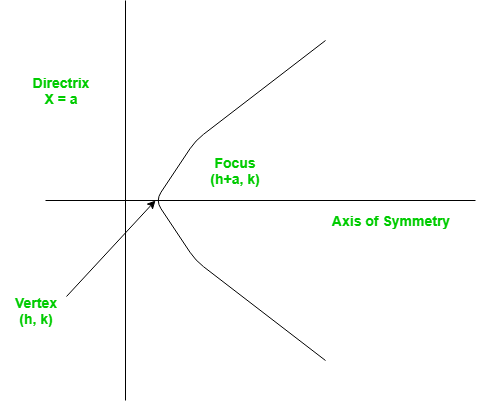Open in App
Not now

# Finding the vertex, focus and directrix of a parabola

• Difficulty Level : Basic
• Last Updated : 22 Jun, 2022

Problem – Find the vertex, focus and directrix of a parabola when the coefficients of its equation are given.
A set of points on a plain surface that forms a curve such that any point on that curve is equidistant from the focus is a parabola.
Vertex of a parabola is the coordinate from which it takes the sharpest turn whereas a is the straight line used to generate the curve.The standard form of a parabola equation is. Given the values of a, b and c; our task is to find the coordinates of vertex, focus and the equation of the directrix.
Example –

```Input : 5 3 2
Output : Vertex:(-0.3, 1.55)
Focus: (-0.3, 1.6)
Directrix: y=-198
Consult the formula below for explanation.```

This problem is a simple example of implementations of formulae. Given below are the required set of formulae which will help us tackle the problem.

`For a parabola in the formVertex:Focus:Directrix:`

## C++

 `#include ` `using` `namespace` `std;`   `// Function to calculate Vertex, Focus and Directrix` `void` `parabola(``float` `a, ``float` `b, ``float` `c)` `{` `    ``cout << ``"Vertex: ("` `<< (-b / (2 * a)) << ``", "` `         ``<< (((4 * a * c) - (b * b)) / (4 * a))` `         ``<< ``")"` `<< endl;` `    ``cout << ``"Focus: ("` `<< (-b / (2 * a)) << ``", "` `         ``<< (((4 * a * c) - (b * b) + 1) / (4 * a))` `         ``<< ``")"` `<< endl;` `    ``cout << ``"Directrix: y="` `         ``<< c - ((b * b) + 1) * 4 * a << endl;` `}`   `// Driver Function` `int` `main()` `{` `    ``float` `a = 5, b = 3, c = 2;` `    ``parabola(a, b, c);` `    ``return` `0;` `}`

## Java

 `// Java program to find the vertex,` `// focus and directrix of a parabola`   `class` `GFG {` `    `  `    ``// Function to calculate Vertex, ` `    ``// Focus and Directrix` `    ``static` `void` `parabola(``float` `a, ` `                         ``float` `b, ``float` `c)` `    ``{` `        `  `        ``System.out.println(``"Vertex: ("` `+` `                          ``(-b / (``2` `* a)) + ``", "` `+` `                          ``(((``4` `* a * c) - (b * b)) /` `                          ``(``4` `* a)) + ``")"``);` `                    `  `        ``System.out.println(``"Focus: ("` `+ ` `                          ``(-b / (``2` `* a)) + ``", "`    `+ ` `                          ``(((``4` `* a * c) - (b * b) + ``1``) /` `                          ``(``4` `* a)) + ``")"``);` `            `  `        ``System.out.println(``"Directrix:"` `+ ``" y="` `+` `                          ``(``int``)(c - ((b * b) + ``1``) * ` `                          ``4` `* a));` `    ``}`   `    ``// Driver Function` `    ``public` `static` `void` `main(String[] args)` `    ``{` `        ``float` `a = ``5``, b = ``3``, c = ``2``;` `        `  `        ``// Function calling` `        ``parabola(a, b, c);` `    ``}` `}`   `// This code is contributed by ` `// Smitha Dinesh Semwal`

## Python 3

 `# Function to calculate Vertex, ` `# Focus and Directrix` `def` `parabola(a, b, c):`   `    ``print``(``"Vertex: ("` `, (``-``b ``/` `(``2` `*` `a)),` `        ``", "``, (((``4` `*` `a ``*` `c) ``-` `(b ``*` `b)) ` `            ``/` `(``4` `*` `a)), ``")"``, sep ``=` `"")` `              `  `    ``print``(``"Focus: ("` `, (``-``b ``/` `(``2` `*` `a)),` `    ``", "``, (((``4` `*` `a ``*` `c) ``-` `(b ``*` `b) ``+` `1``)` `            ``/` `(``4` `*` `a)), ``")"``, sep ``=` `"")` `               `  `    ``print``(``"Directrix: y="``, c ``-` `((b ``*` `b)` `                ``+` `1``) ``*` `4` `*` `a, sep ``=` `"")`   `# Driver Function` `a ``=` `5` `b ``=` `3` `c ``=` `2` `parabola(a, b, c)`   `# This code is contributed by Smitha.`

## C#

 `// C# program to find the vertex,` `// focus and directrix of a parabola` `using` `System;`   `class` `GFG {` `    `  `    ``// Function to calculate Vertex, ` `    ``// Focus and Directrix` `    ``static` `void` `parabola(``float` `a, ` `                         ``float` `b, ``float` `c)` `    ``{` `        ``Console.WriteLine(``"Vertex: ("` `+` `                         ``(-b / (2 * a)) + ``", "` `+` `                         ``(((4 * a * c) - (b * b)) /` `                         ``(4 * a)) + ``")"``);` `                    `  `        ``Console.WriteLine(``"Focus: ("` `+` `                         ``(-b / (2 * a)) + ``", "` `+` `                         ``(((4 * a * c) - (b * b) + 1) /` `                         ``(4 * a)) + ``")"``);` `                `  `        ``Console.Write(``"Directrix:"` `+ ``" y="` `+ ` `                     ``(``int``)(c - ((b * b) + 1) * 4 * a));` `    ``}`   `    ``// Driver Function` `    ``public` `static` `void` `Main()` `    ``{` `        ``float` `a = 5, b = 3, c = 2;` `        `  `        ``// Function calling` `        ``parabola(a, b, c);` `    ``}` `}`   `// This code is contributed by nitin mittal`

## PHP

 ``

## Javascript

 ``

Output –

```Vertex:(-0.3, 1.55)
Focus: (-0.3, 1.6)
Directrix: y=-198```

Time Complexity: O(1)

Auxiliary Space: O(1)

My Personal Notes arrow_drop_up
Related Articles